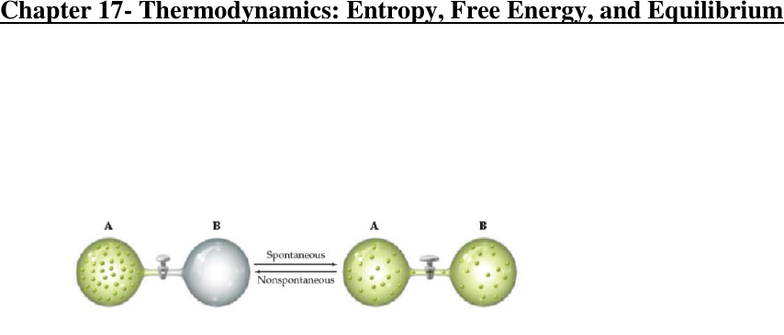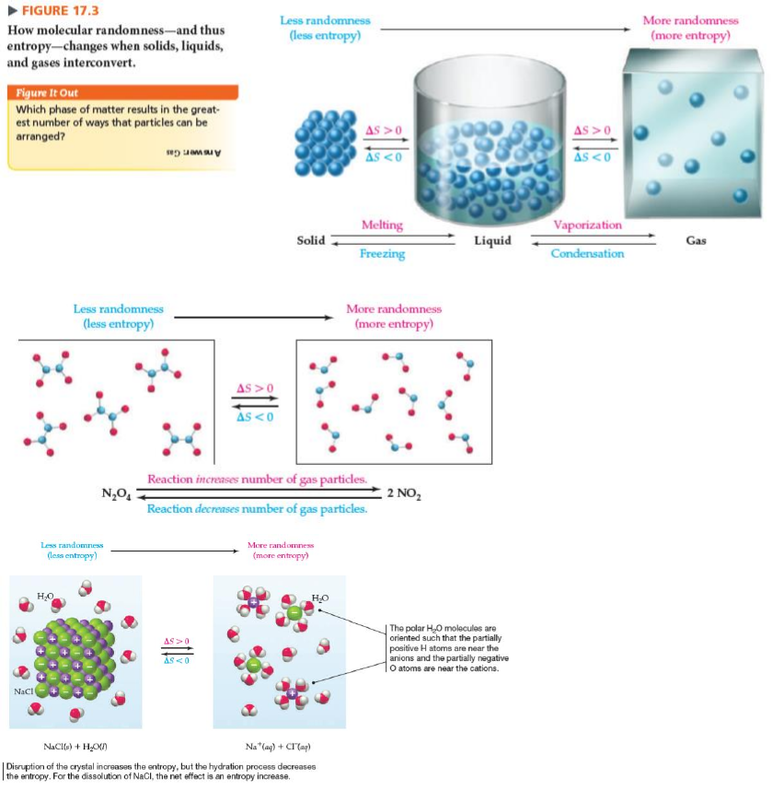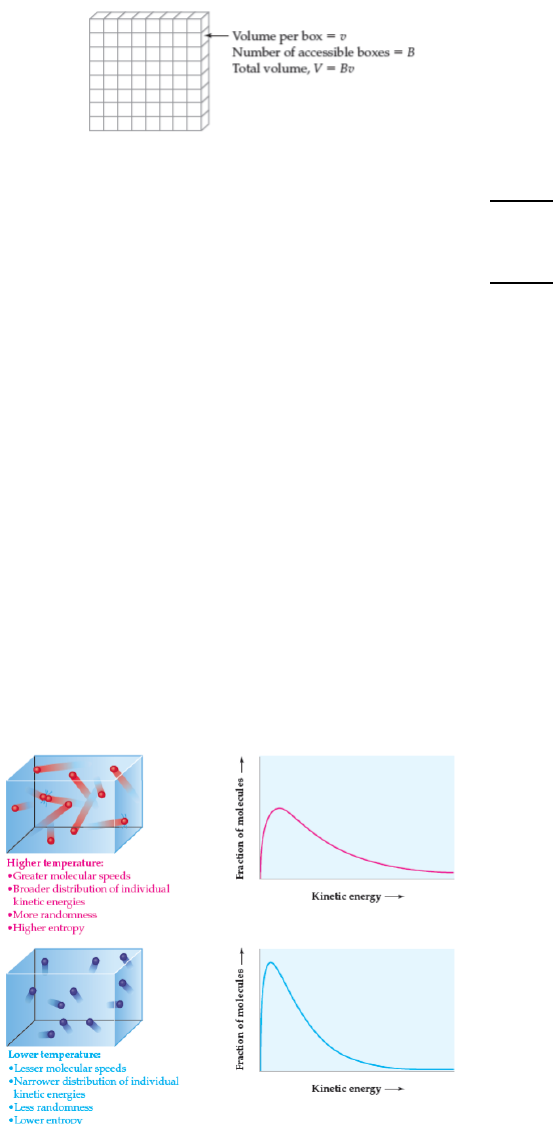# CHM135H1 Chapter Notes - Chapter 17: Spontaneous Process, Stopcock, Gas Constant

138 views8 pages
School
Department
CourseCHM135 CHEMISTRY TEXTBOOK CH. 17 NOTES/SUMMARY
Chapter 17- Thermodynamics: Entropy, Free Energy, and Equilibrium
Chapter 17.1: Spontaneous Processes
- E.g. Expansion of a gas into a vacuum when a stopcock inbetween two bulbs of gas is opened,
gas in bulb A expands spontaneously into evacuated bulb B until gas pressure in two bulbs is the
same; revers process of migrations of all gas molecules into one bulb, does not occur
spontaneously to compress a gas from a larger to a smaller volume, gas needs to be pushed on
with a piston
(Textbook, Page 716)
- A spontaneous reaction always moves a reaction mixture toward equilibrium a
nonspontaneous reaction moves the composition of a mixture away from the equilibrium
composition
- Thermodynamics tells us where a reaction is headed but not how long it takes to get there a
spontaneous reaction occurs slowly if it has a high activation energy Ea
-
Chapter 17.2: Enthalpy, Entropy, and Spontaneous Processes: A Brief Review
- Since some spontaneous reactions are exothermic and others are endothermic, enthalpy alone
can’t account for the direction of spontaneous change; a second factor must be involved
nature’s tendency to move to a condition of maximum randomness
- Molecular randomness is called entropy and is denoted by the symbol S
- Entropy is a state function and the entropy change ΔS for a process depends only on initial and
final state of the system
- Randomness of a system comes about because the particles in the system (atoms, ions, and
molecules) are in constant motion, moving about in accessible volume, colliding with each other
and continually exchanging energy
- Randomness and entropy is a probability concept, related to number of ways that a particular state
of a system can be achieved
- Particular state of a macroscopic system, characterized by temperature, pressure, volume, and
number of particles, can be achieved by methods in which fluctuating positions and energies of
individual particles differ but volume and total energy are constant
Unlock document

This preview shows pages 1-3 of the document.
Unlock all 8 pages and 3 million more documents.-
(Textbook, Page 718)
- (Textbook, Page 719)
- (Textbook, Page 719)
Chapter 17.3: Entropy and Probability
- Why do systems tend to move spontaneously to a state of minimum randomness?
o Random arrangement of particles is more probable than an ordered arrangements because
a random arrangement can be achieved in more ways
- Boltzmann’s Equation
o  ; where S is the entropy of the state, ln W is the natural logarithm of the
number of ways that the state can be achieved, and k is Boltzmann’s constant (k = R/NA
= 1.38 x 10-23) entropy has same units as constant k, J/K
o Entropy associated with a random arrangement of molecules in space is soemtiems called
positional, or configurational, entropy
- Expansion of an ideal gas
o Boltzmann’s equation also explains why a gas expands in a vacuum a gas expands
spontaneously because the state of greater volume is more probable
o Since the energy of an ideal gas depends only on the temperature, ΔE or the expansion of
an ideal gas at constant temperature is zero
Unlock document

This preview shows pages 1-3 of the document.
Unlock all 8 pages and 3 million more documents.o The number of ways that N molecules can occupy B boxes is W = BN
(Textbook, Page 722)
o Entropy change upon volume change of a gas:
   


o Entropy change upon pressure change of a gas:
   

o The entropy of a gas increases when its pressure decreases at constant temperature, and
the entropy decreases when its pressure increases the more gas is compressed, the less
space the gas molecules have and so randomness decreases
Chapter 17.4: Entropy and Temperature
- Entropy is associated with the orientation and distribution of molecules in space
- Disordered crystals have higher entropy than ordered crystals and expanded gases have higher
entropy than compressed gases
- Entropy is also associated with molecular motion as temperature of a substance increases,
random molecular motion increases and there is a corresponding increase in the average kinetic
energy of the molecules; not all gases have the same energy
- In solids, liquids and gases, the total energy of a substance can be distributed among the
individual molecules in a number of ways that increases as the total energy increases
- According to Boltzmann’s equation, the more ways that the energy can be distributed, the greater
the randomness of the state and the higher its entropy
- Entropy of a substance increases with increasing temperature
- (Textbook, Page 724)
Unlock document

This preview shows pages 1-3 of the document.
Unlock all 8 pages and 3 million more documents.

# Get access

\$10 USD/m
Billed \$120 USD annually
Homework Help
Class Notes
Textbook Notes